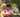### JavaScript Functions

December 03, 2019

Source

JavaScript functions are defined with the function keyword. You can use a function declaration or a function expression.

Declared functions are not executed immediately. They are "saved for later use", and will be executed later, when they are invoked (called upon).

``````function myFunction(a, b) {
return a * b;
}``````

Semicolons are used to separate executable JavaScript statements. Since a function declaration is not an executable statement, it is not common to end it with a semicolon.

A JavaScript function can also be defined using an expression. A function expression can be stored in a variable:

``var x = function (a, b) {return a * b};``

After a function expression has been stored in a variable, the variable can be used as a function:

``````var x = function (a, b) {return a * b};
var z = x(4, 3);``````

The function above is actually an anonymous function (a function without a name). Functions stored in variables do not need function names. They are always invoked (called) using the variable name. The function above ends with a semicolon because it is a part of an executable statement.

###Self-Invoking Functions###

Function expressions can be made "self-invoking". A self-invoking expression is invoked (started) automatically, without being called. Function expressions will execute automatically if the expression is followed by (). You cannot self-invoke a function declaration. You have to add parentheses around the function to indicate that it is a function expression:

``````(function () {
var x = "Hello!!";  // I will invoke myself
})();``````

The function above is actually an anonymous self-invoking function (function without name)

Functions are Objects

The typeof operator in JavaScript returns "function" for functions. But, JavaScript functions can best be described as objects. JavaScript functions have both properties and methods. The arguments.length property returns the number of arguments received when the function was invoked:

``````function myFunction(a, b) {
return arguments.length;
}``````

The toString() method returns the function as a string:

``````function myFunction(a, b) {
return a * b;
}

var txt = myFunction.toString();``````

###Arrow Functions###

Arrow functions allows a short syntax for writing function expressions. You don't need the function keyword, the return keyword, and the curly brackets.

``````// ES5
var x = function(x, y) {
return x * y;
}

// ES6
const x = (x, y) => x * y;``````

Arrow functions are not hoisted. They must be defined before they are used. Using const is safer than using var, because a function expression is always constant value. You can only omit the return keyword and the curly brackets if the function is a single statement. Because of this, it might be a good habit to always keep them:

``const x = (x, y) => { return x * y };``Posted by Tai Lu ( 呂台生 ) who's building useful things.You should follow him on Twitter